Vectors and conics

This free course is available to start right now. Review the full course description and key learning outcomes and create an account and enrol if you want a free statement of participation.

Free course

4.9 Further exercises

Example 58

Determine the equation of the circle with centre (2, 1) and radius 3.

The equation of the circle is

(x − 2)2 + (y − 1)2 = 32,

which can be rewritten in the form

x2 + y2 − 4x − 2y − 4 = 0.

Example 59

Determine the points of intersection of the line with equation y = x + 2 and the circle in Exercise 58.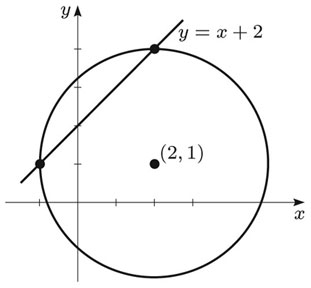At the points of intersection of the line and the circle, the following equations must be satisfied:

y = x + 2

and

x2 + y2 − 4x − 2y − 4 = 0.

Substituting the expression for y from the first equation into the second equation, we obtain

x2 + (x + 2)2 − 4x − 2(x + 2) − 4 = 0.

Multiplying this out and collecting terms, we obtain

2x2 − 2x − 4 = 0,

or

x2 − x − 2 = 0.

This can be factorised as

(x − 2)(x + 1) = 0,

so x = 2 or x = −1.

Substituting these values of x into the equation y = x + 2 of the line, we find that the corresponding values of y are y = 4 and y = 1.

Hence the points of intersection of the line and the circle are (2, 4) and (−1, 1).

Example 60

This question concerns the parabola y2 = 4ax (a > 0) with parametric equations x = at2, y = 2at and focus F. Let P and Q be points on the parabola with parameters t1 and t2, respectively. Prove that:

• (a) if PQ subtends a right angle at the vertex O of the parabola, then t1t2 = −4;

•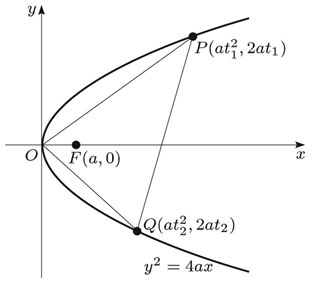• (b) if t1 = 2 and PQ is perpendicular to FP, then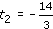.

• (a) The gradient of OP is

•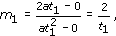• and the gradient of OQ is

•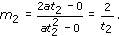• OP and OQ are perpendicular (in other words, PQ subtends a right angle at O) if m1m2 = −1, that is, if

•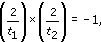• which reduces to t1t2 = −4, as required.

• (b) Here P is the point (4a, 4a). Also, F is (a, 0), so the gradient of FP is

•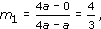• and the gradient of PQ is

•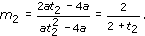• FP and PQ are perpendicular if m1m2 = −1, that is, if

•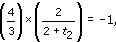• which reduces to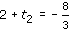, so, as required.

Example 61

This question concerns the rectangular hyperbola xy = c2 (c > 0) with parametric equations x = ct, y = c/t. Let P and Q be points on the hyperbola with parameters t1 and t2, respectively.

• (a) Determine the equation of the chord PQ.

•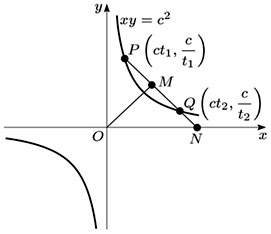• (b) Determine the coordinates of the point N where PQ meets the x-axis.

• (c) Determine the midpoint M of PQ.

• (d) Prove that OM = MN, where O is the origin.

• (a) The gradient of the chord PQ is

•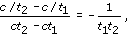• so the equation of PQ is

•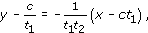• that is,

•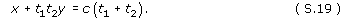• (b) At the point N where PQ meets the x-axis, y = 0 and equation (S.19) also holds, so x = c(t1 + t2). Hence N is the point with coordinates (c(t1 + t2), 0).

• (c) The midpoint M of PQ is

•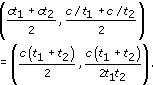• (d) By the Distance Formula, the distance OM is

•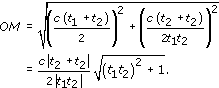• Also,

•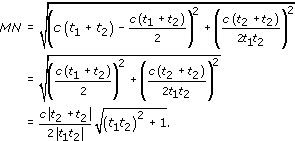• Thus OM = MN.

• Remark: An alternative way of proving part (d) is as follows: Since the point M is vertically above the midpoint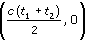of ON, the triangle OMN must be an isosceles triangle, so OM = MN.

M208_1